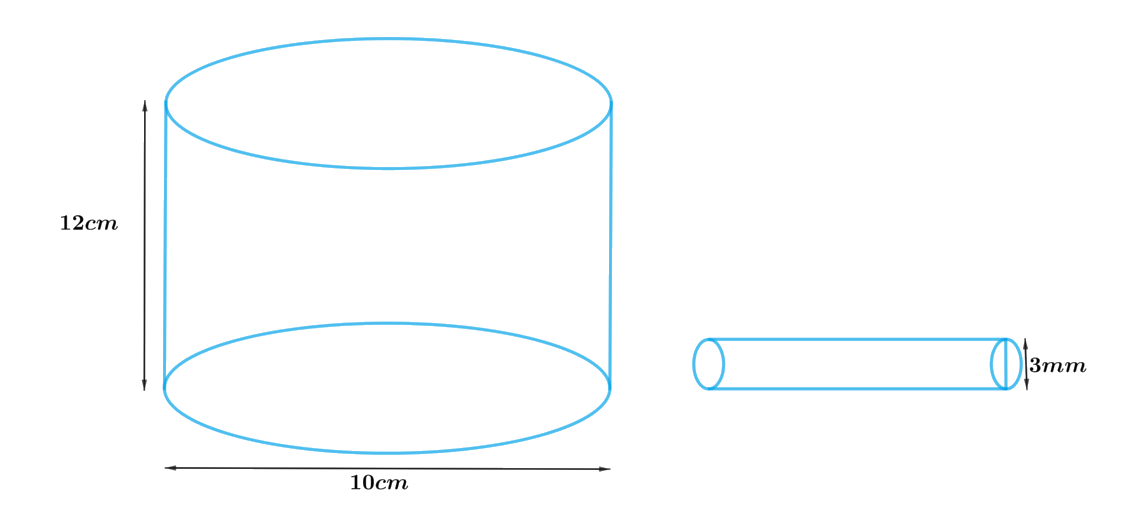# Ex.13.5 Q1 Surface Areas and Volumes Solution - NCERT Maths Class 10

Go back to  'Ex.13.5'

## Question

A copper wire, $$3\,\rm{mm}$$ in diameter, is wound about a cylinder whose length is $$12\,\rm{cm}$$, and diameter $$10\,\rm{cm}$$, so as to cover the curved surface of the cylinder. Find the length and mass of the wire, assuming the density of copper to be $$8.88\,\rm{g\,per}$$ $$\rm{cm^3}$$

Video Solution
Surface Areas And Volumes
Ex 13.5 | Question 1

## Text Solution

What is Known?

Diameter of the copper wire is $$3\rm{mm.}$$ Length of the cylinder is $$12\rm{cm}$$ and diameter is $$10\rm{cm.}$$ Density of copper is $$8.88 \,\,\text{g per} \,\rm{cm^3}$$

What is Unknown?

Length and mass of the wire

Reasoning:

Draw a figure to visualize the cylinderTo find length of the wire:

Since the curved surface of the cylinder to be covered with the copper wire then one round of copper wire will cover same height of cylinder as of the width (diameter) of the wire.

Therefore, Length of the wire $$\times$$ diameter the of wire $$=$$ CSA of the cylinder

We will find the CSA of the cylinder by using formula;

CSA of the cylinder $$= 2\pi rh$$

where $$r$$ and $$h$$ are radius and height of the cylinder respectively.

To find mass of the wire:

Mass $$=$$ Density $$\times$$ Volume

As the density of the copper is given, we need to find volume of the wire first.

Since the wire is cylindrical in shape

Therefore, Volume of the wire $$=$$ volume of the cylinder

We will find the volume of the cylindrical wire by using formula;

Volume of the cylinder $$= \pi {r_1}^2{h_1}$$

where $$r1$$ and $$h1$$ are the radius and the length of the cylindrical wire

Steps:

Height of cylinder,$$h = 12cm$$

Diameter of cylinder,$$d = 10cm$$

Radius of cylinder,\begin{align}r = \frac{{10cm}}{2} = 5cm\end{align}

Diameter (width) of wire\begin{align}{d_1} = 3mm = \frac{3}{{10}}cm = 0.3cm\end{align}

Radius of wire\begin{align}{r_1} = \frac{{0.3cm}}{2} = 0.15cm\end{align}

Let the length of the wire be $$h1$$

Since curved surface of the cylinder to be covered with the wire

Length of the wire $$\times$$ diameter the of wire $$=$$ CSA of the cylinder

\begin{align}{d_1} \times {h_1} &= 2\pi rh\\{h_1} &= \frac{{2\pi rh}}{{{d_1}}}\\&= \frac{{2 \times 3.14 \times 5cm \times 12cm}}{{0.3cm}}\\&= 1256cm\end{align}

Volume of the wire $$=$$ volume of the cylinder

\begin{align} & =\pi {{r}_{1}}^{2}{{h}_{1}} \\ & =\left[ \begin{array} & 3.14\times 0.15cm\times \\ 0.15cm\times 1256cm \\ \end{array} \right] \\ & =88.7364c{{m}^{3}} \\\end{align}

Density of copper$$= 8.8g/c{m^3}$$

Mass of the copper Wire $$=$$ Density $$\times$$ volume

\begin{align}&= 8.88g/c{m^3} \times 88.7364c{m^3}\\&= 787.97923g\end{align}

Length of the wire is $$1256cm$$ and Mass is $$788g{\rm{ }}$$ (approx.)

Learn from the best math teachers and top your exams

• Live one on one classroom and doubt clearing
• Practice worksheets in and after class for conceptual clarity
• Personalized curriculum to keep up with school